##### Geometry For DummiesIf you know the base and height of a triangle, you can use a tried-and-true formula to find its area. You likely first ran into the basic triangle area formula in about sixth or seventh grade. If you’ve forgotten it, no worries — here it is: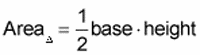Assume for the sake of argument that you have trouble remembering this formula. Well, you won’t forget it if you focus on why it’s true. So why does the area of a triangle equal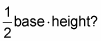Because the area of a rectangle is base times height (which is the same thing as length times width), and a triangle is half of a rectangle.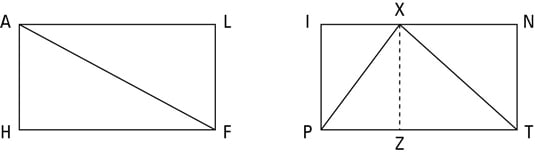Check out the above figure, which shows two triangles inscribed in rectangles HALF and PINT.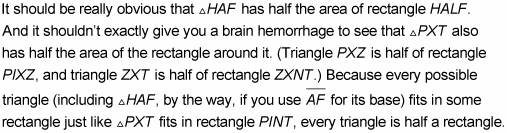Now for a problem that involves finding the area of a triangle: What’s the length of altitude segment XT in triangle WXR in the following figure?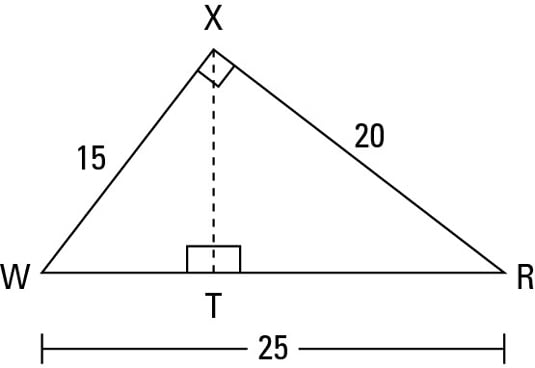The trick here is to note that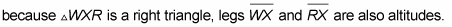Thus, you can use either leg as the altitude, and then the other leg automatically becomes the base. Plug their lengths into the formula to determine the triangle’s area: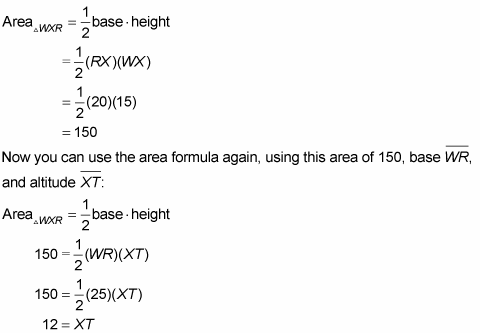Bingo!